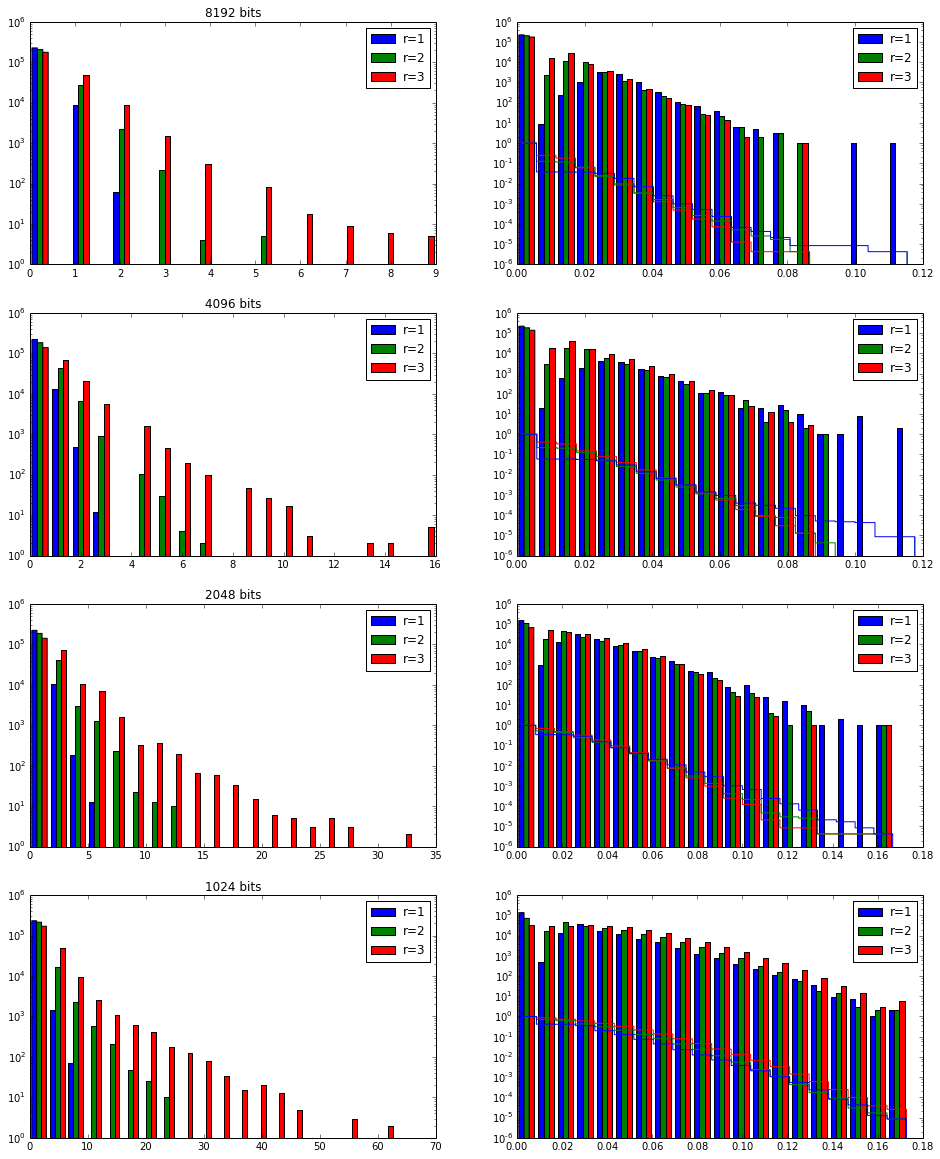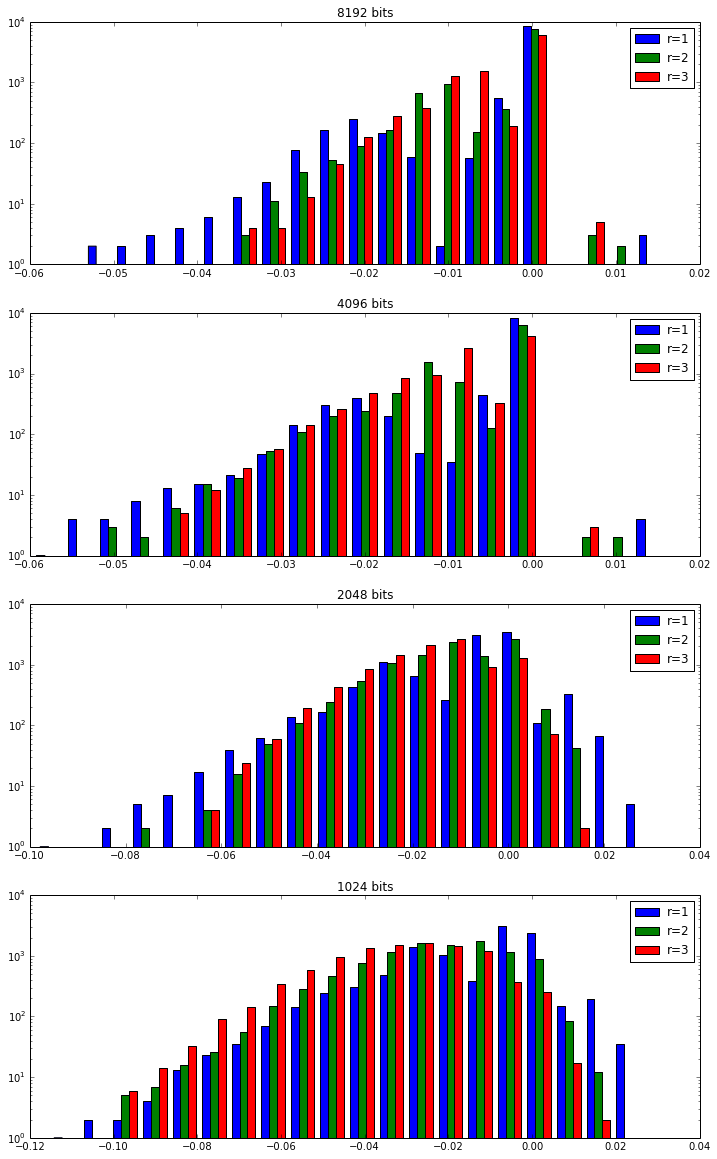# Looking at bit collisions in Morgan fingerprints

In the group over the past few days we've had a few conversations about bit collisions in Morgan fingerprints. These happen when we fold the fingerprints to be a particular size if two different bits (corresponding to two different atom environments) end up folding onto the same bit.

This post is an exploration of how often that happens. I will look at fingerprints with different radii -- 1, 2, and 3 -- folded to a set of different sizes -- 1K, 2K, 4K, 8K.

TL;DR version: There are a fair number of collisions at fingerprint sizes below 4K. As expected, higher radii have more collisions. The collisions don't end up making much difference in terms of calculated similarity, but we have observed (not shown here) that it can make a measurable difference in the performance of some machine-learning algorithms.

In :
```from rdkit import Chem,DataStructs
import time,random
import numpy as np
from collections import defaultdict
from rdkit.Chem import Draw
from rdkit.Chem import rdMolDescriptors as rdmd
from rdkit.Chem.Draw import IPythonConsole
from rdkit import DataStructs
from rdkit import rdBase
from __future__ import print_function

print(rdBase.rdkitVersion)
import time
print(time.asctime())
```
```2014.03.1pre
Fri Feb 28 07:44:17 2014

```

For test data I'll use the ChEMBL compounds from 2010-2012 that I built in an earlier post. This is a set of about 230K compounds.

In :
```filen='../data/chembl16_2010-2012.smi'
!wc -l \$filen
!head \$filen
```
```  234681 ../data/chembl16_2010-2012.smi
Br\C=C\1/CCC(C(=O)O1)c2cccc3ccccc23 23
COc1cc2nc(nc(N)c2cc1OC)N3CCN(CC3)C(=O)c4occc4 97
CN1CCC[C@H]1c2cccnc2 115
CC1COc2c(N3CCN(C)CC3)c(F)cc4C(=O)C(=CN1c24)C(=O)O 146
CCN1C=C(C(=O)O)C(=O)c2ccc(C)nc12 147
Oc1cc2C(=O)Oc3c(O)c(O)cc4C(=O)Oc(c1O)c2c34 148
COc1ccc2c(c1)c(CC(=O)O)c(C)n2C(=O)c3ccc(Cl)cc3 173
CC1(C)[C@@H](N2[C@@H](CC2=O)S1(=O)=O)C(=O)O 194
Cn1cc(C2=C(C(=O)NC2=O)c3cn(CCCSC(=N)N)c4ccccc34)c5ccccc15 213
CN1CCN(CC1)c2cc3N(C=C(C(=O)O)C(=O)c3cc2F)c4ccc(F)cc4 205

```

Loop over the molecules and build fingerprints of multiple radii and folded lengths.

In :
```counts=defaultdict(list)
for i,line in enumerate(file(filen)):
m = Chem.MolFromSmiles(line.split())
if not m: continue
for v in 1,2,3:
counts[(v,-1)].append(len(rdmd.GetMorganFingerprint(m,v).GetNonzeroElements()))
for l in 1024,2048,4096,8192:
counts[(v,l)].append(rdmd.GetMorganFingerprintAsBitVect(m,v,l).GetNumOnBits())
if not (i+1)%5000:
print("Done {0}".format(i+1))
```
```Done 5000
Done 10000
Done 15000
Done 20000
Done 25000
Done 30000
Done 35000
Done 40000
Done 45000
Done 50000
Done 55000
Done 60000
Done 65000
Done 70000
Done 75000
Done 80000
Done 85000
Done 90000
Done 95000
Done 100000
Done 105000
Done 110000
Done 115000
Done 120000
Done 125000
Done 130000
Done 135000
Done 140000
Done 145000
Done 150000
Done 155000
Done 160000
Done 165000
Done 170000
Done 175000
Done 180000
Done 185000
Done 190000
Done 195000
Done 200000
Done 205000
Done 210000
Done 215000
Done 220000
Done 225000
Done 230000

```

Now plot histograms of the numbers of collisions along with the fractions of collisions.

The two plots in each row show the same data, the left one uses counts of collisions, the right is the fraction of bits that are collisions. The right plots also include lines showing what fraction of fingerprints have at least that fraction of collisions.

In :
```figure(figsize=(16,20))

pidx=1
#----------------------------
for nbits in (8192,4096,2048,1024):
subplot(4,2,pidx)
pidx+=1
v1=np.array(counts[1,-1])
v2=np.array(counts[1,nbits])
d1 = v1-v2
d1p=np.array(d1,np.float)
d1p/=v1
v1=np.array(counts[2,-1])
v2=np.array(counts[2,nbits])
d2 = v1-v2
d2p=np.array(d2,np.float)
d2p/=v1
v1=np.array(counts[3,-1])
v2=np.array(counts[3,nbits])
d3 = v1-v2
d3p=np.array(d3,np.float)
d3p/=v1

_=hist((d1,d2,d3),bins=20,log=True,label=("r=1","r=2","r=3"))
title('%d bits'%nbits)
_=legend()

subplot(4,2,pidx)
pidx+=1
_=hist((d1p,d2p,d3p),bins=20,log=True,label=("r=1","r=2","r=3"))
_=hist((d1p,d2p,d3p),bins=20,histtype='step',cumulative=-1,normed=True, color=['b','g','r'])
_=legend()
```So, there are definitely some collisions.

Do they make a difference in similarity values? Look at 10K random molecule pairs to find out.

In :
```random.seed(0xF00D)
smis = [x.split() for x in file(filen)]
ivs=[random.randint(0,len(smis)-1) for x in range(10000)]
jvs=[random.randint(0,len(smis)-1) for x in range(10000)]
pairs=zip(ivs,jvs)

sims=defaultdict(list)
for i,j in pairs:
mi = Chem.MolFromSmiles(smis[i])
if not mi:
continue
mj = Chem.MolFromSmiles(smis[j])
if not mj:
continue
for r in 0,1,2,3:
fpi=rdmd.GetMorganFingerprint(mi,r)
for k,v in fpi.GetNonzeroElements().items():
fpi[k]=1
fpj=rdmd.GetMorganFingerprint(mj,r)
for k,v in fpj.GetNonzeroElements().items():
fpj[k]=1
sims[(r,-1)].append(DataStructs.TanimotoSimilarity(fpi,fpj))
for l in 1024,2048,4096,8192:
fpi=rdmd.GetMorganFingerprintAsBitVect(mi,r,l)
fpj=rdmd.GetMorganFingerprintAsBitVect(mj,r,l)
sims[(r,l)].append(DataStructs.TanimotoSimilarity(fpi,fpj))
```
In :
```figure(figsize=(12,20))

pidx=1
#----------------------------
for nbits in (8192,4096,2048,1024):
subplot(4,1,pidx)
pidx+=1
v1=np.array(sims[1,-1])
v2=np.array(sims[1,nbits])
d1 = v1-v2
d1p=np.array(d1,np.float)
d1p/=v1
v1=np.array(sims[2,-1])
v2=np.array(sims[2,nbits])
d2 = v1-v2
d2p=np.array(d2,np.float)
d2p/=v1
v1=np.array(sims[3,-1])
v2=np.array(sims[3,nbits])
d3 = v1-v2
d3p=np.array(d3,np.float)
d3p/=v1

_=hist((d1,d2,d3),bins=20,log=True,label=("r=1","r=2","r=3"))
title('%d bits'%nbits)
_=legend()

```
```-c:12: RuntimeWarning: invalid value encountered in divide
-c:17: RuntimeWarning: divide by zero encountered in divide
-c:17: RuntimeWarning: invalid value encountered in divide
-c:22: RuntimeWarning: divide by zero encountered in divide
-c:22: RuntimeWarning: invalid value encountered in divide
-c:12: RuntimeWarning: divide by zero encountered in divide

```The changes in similarity are very minimal.

In []: